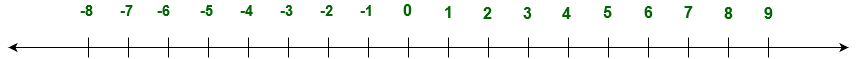# GRE Arithmetic | Integers

• Last Updated : 23 Aug, 2021

There is a different type of numbers: natural number, real number, whole number, integers, etc. Integers are the numbers -1, -2, -3, -4, 1, 2, 5, 9, -10. Integers are either negative or positive (0 is the exception which is neither negative nor positive integer).

Let’s understand it by number line:Types of integers:

• Positive integer (e.g. 5, 8, 12, 29 etc).
• Negative integer (e.g. -5, -8, -12, -29 etc).
• Even integers (e.g. 4, 8, 12, 28, -28, -12, -8, -4 etc).
• Odd integers (e.g. 5, 9, 13, 29, -33. -31, -49 etc).
• Neither positive nor negative integer is 0.
• Prime number is an integer which is divisible by only two positive integers (1 and the integer itself, e.g. 2, 3, 5, 7, 11, 29, etc).
• Coprime number is pair of two positive integers which have 1 as a only common positive divisor.

What is least common multiple and greatest common divisor?

Least common divisor (LCM): It is the least common multiple of two positive integers.

```Example:
6 is the LCM of 2 and 3 ```

Greatest common divisor (GCD): It is the greatest common factor (GCF) of two positive integers.

```Example:
GCD of 15 and 30 is 15 ```

Different type of operation can be performed on integers:

1. Addition of two integers will be an integer (negative or positive).
2. Subtraction of two integers will be an integer (negative or positive).
3. Product of two integers will be an integer (negative or positive).
4. Division of two integers can be an integer or decimal (negative or positive).
5. Square of an integer (negative or positive) will be a positive integer.
6. Root of a positive integer can be an integer or decimal.
```odd + odd = even
odd - odd = even
even + even = even
even - even = even
odd + even = odd
odd - even = even
Even - odd = odd
odd * odd = odd
even * even = even
odd * even = even ```

For example:

```3 + 3 = 6
(-3) + (-3) = -6
3 - 1 = 2
(-3) - (-1) = -2
4 + 4 = 8
(-4) + (-4) = -8
4 - 2 = 2
(-4) - (-2) = (-2)
3 + 4 = 7
(-3) + (-4) = (-7)
5 - 2 = 3
(-5) - (-2) = (-3)
5 - 3 = 2
(-5) - (-3) = (-2)
3 * 3 = 9
(-3) * (-3) = 9
4 * 4 = 16
(-4) * (-4) = 16
3 * 4 = 12
(-3) * (-4) = 12
√4 = 2
√2 = 1.41421...
6 / 2 = 3
(-6) / (-2) = 3
(-6) / 2 = -3
6 / (-2) = -3
5 / 2 = 2.5
(-5) / (-2) = 2.5
5 / (-2) = -2.5
(-5) / 2 = -2.5```

My Personal Notes arrow_drop_up